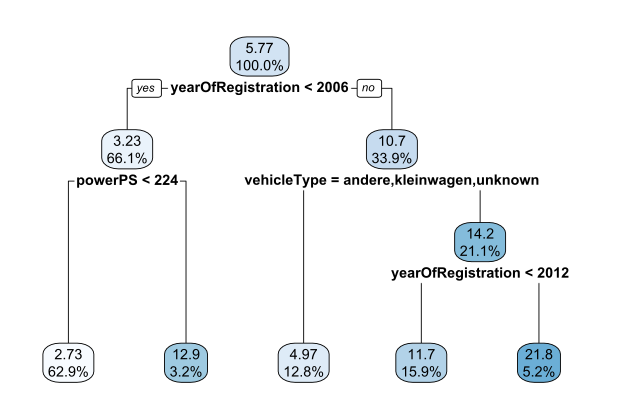Videos and questions for Chapter 2b of the course "Market Analysis with Econometrics and Machine Learning" at Ulm University (taught by Sebastian Kranz)

### Regression Trees

Take a look at the estimated regression tree from our slides:What is the predicted price (in 1000 Euro) for a car registered in 2005 with a horse power of 250?

What is the share of cars registered before 2006?

What is the share of cars with fewer than 224 PS?

### Estimating a regression tree

Remark: Take a look at the lecture slides to see how a split for a nominal variable is computed.

### Random forests

What are the random elements in a random forest? (Make a guess)
A: Each tree is estimated with a data set that is randomly drawn with replacement from the training data set.
B: We estimate several trees but pick a random subset of trees for the prediction.
C: When training a tree, at each node only a random subset of variables is considered for the optimal split.

### Heterogenous Treatment Effects and Causal Forests

Assume we want to estimate heterogenoues treatments effects for males and females by estimating the following regression with interaction effects (recall Chapter 1c):

$y_i = \beta_0 + \beta_1 w_i + \beta_2 female_i + \beta_3 female_i \cdot w_i + \varepsilon$

What would then be the estimated treatment effect for females?

Assume we would run a lasso regression of the form

$y_i = \beta_0 + \beta_1 w_i + \beta_2 female_i + \beta_3 female_i \cdot w_i + ... + \varepsilon$ where the ... describes many more explanatory variables and interaction effects with the treatment indicator to account for heterogenous effects in many potentially relevant subgroups.

We know that a lasso regression can select relevant predictors and set other coefficients to 0. Would the selected interaction terms from running the lasso regression above yield relevant subgroups that have strong or weak treatment effects?

### Causal Tree

Assume that in a particular leaf of a causal forest, we have the following training data observations:

 w y 0 10 0 12 1 5 1 7 1 3

What would then the predicted value for an observation falling into this leaf?

### Training a causal tree

Does the fact that honest predictions use only half of the observations in a tree make the predictions of a causal forest less precise compared let's say to a random forest?

### Discussion: Searching for Subgroups and Endogeneity Problems

That's all. So it's a good time to start the RTutor problem set...